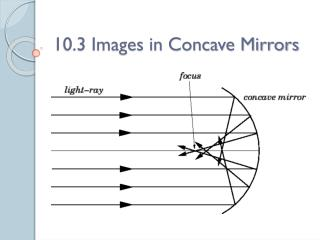DownloadDownload Presentation10.3 Images in Concave Mirrors

# 10.3 Images in Concave Mirrors

Download Presentation## 10.3 Images in Concave Mirrors

- - - - - - - - - - - - - - - - - - - - - - - - - - - E N D - - - - - - - - - - - - - - - - - - - - - - - - - - -
##### Presentation Transcript

1. 10.3 Images in Concave Mirrors

2. Concave Mirror • Unlike a plane mirror, a curved mirror produces an image that is a different size, shape, and/or orientation. • Imagine remove a square out of a basketball and covering this region with a mirror • The inner region produces a concave mirror

3. Images in a Concave Mirror • In a concave mirror, the images towards the edges of the mirror produce increasingly more distorted images

4. Drawing Ray Diagrams for Concave Mirrors • When dealing with a curved mirror, you should treat each curved surface as many small, flat mirrors (Figure 10.20A). • Each of the normal’s (in blue) intersect at a point called the centre of curvature (c)

5. Draw Ray Diagrams for Concave Mirrors • The thick horizontal normal that touches the centre of the mirror is called the principal axis. • The point at which the principal axis cuts the centre of the mirror is called the vertex (V). principal axis. V

6. Draw Ray Diagrams for Concave Mirrors • If an incident ray passes through the centre of curvature, the ray will reflect back onto itself. • The angle of incidence is zero, therefore the angle of reflection is zero • This holds true for all rays passing through point “c”. V

7. Draw Ray Diagrams for Concave Mirrors • An incident ray that is near and parallel to the principal axis will reflect and intersect at the focal point (F). • All incidence rays that are near and parallel to the principal axis will reflect through point “F”. • Any rays that pass through point “F” will reflect and travel parallel to the principal axis. V

8. Draw Ray Diagrams for Concave Mirrors • The distance between the focal point (f) and the vertex (v) is called the focal length. V

9. Drawing an object between the focal point and the mirror • When an object is placed between the focal point and the mirror, the image produced is an uprightvirtual image that is enlarged. • Take 8 minutes to follow all the steps on page 422. • You may be asked to come to the board and complete one of these steps

10. An object between the focal point and the centre of curvature • When an object is between the focal point and the centre of curvature, the image produced is an invertedreal image that is enlarged. • Take 8 minutes to follow all the steps on page 423. • You may be asked to come to the board and complete one of these steps

11. An object beyond the centre of curvature • When an object is beyond the centre of curvature, the reflection is smaller and inverted as a real image. • Follow page 424 and draw the reflection for an object that is beyond the centre of curvature.

12. Mirror and Magnification Equations • The characteristics of an image can also be predicted by using two equations: • Mirror Equation: • Allows us to determine the focal point, distance of the image, or the distance of the object • Must know two of the three variables in order to solve the third • Magnification Equation: • Allows us to determine the height of the object or the height of the image. • This equation is usually used following the mirror equation

13. Mirror Equation • The mirror equation allows you to calculate the location of an image • The mirror equation is seen below • do represents the distance of the object • direpresents the distance of the image • f represents focal length

14. Mirror Equation • If the image distance di is negative, then the image is behind the mirror (a virtual image)

15. Mirror Equation Question • A concave mirror has a focal length of 12 cm. An object with a height of 2.5 cm is placed 40 cm in front of the mirror. • Calculate the image distance. • Is the image in front of the mirror or behind? How do you know this?

16. Magnification Equation • The magnification (m) tells you the size, or height of the image relative to the object, using object and image distances. • Therefore, in order to use this equation the distance of the object and image must be known.

17. Magnification Equation • If the image height hi is negative, the image is inverted relative to the object.

18. Magnification Equation Question • A concave mirror has a focal length of 12 cm. An object with a height of 2.5 cm is placed 40 cm in front of the mirror. The image distance has been calculated to be 17.14 cm. • What is the height of the image? • Is the image inverted? Explain.

19. Additional Problems • Questions 1-5 on page 427 • Questions 1-8 on page 430